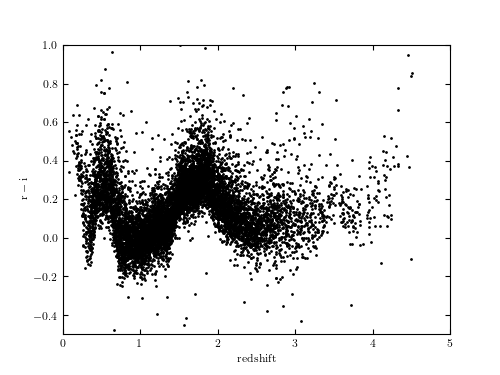# SDSS DR7 Quasars¶

Figure 1.4.

The r-i color vs. redshift diagram for the first 10,000 entries from the SDSS Data Release 7 Quasar Catalog. The color variation is due to emission lines entering and exiting the r and i band wavelength windows.# Author: Jake VanderPlas
#   The figure produced by this code is published in the textbook
#   "Statistics, Data Mining, and Machine Learning in Astronomy" (2013)
#   To report a bug or issue, use the following forum:
from matplotlib import pyplot as plt
from astroML.datasets import fetch_dr7_quasar

#----------------------------------------------------------------------
# This function adjusts matplotlib settings for a uniform feel in the textbook.
# Note that with usetex=True, fonts are rendered with LaTeX.  This may
# result in an error if LaTeX is not installed on your system.  In that case,
# you can set usetex to False.
if "setup_text_plots" not in globals():
from astroML.plotting import setup_text_plots
setup_text_plots(fontsize=8, usetex=True)

#------------------------------------------------------------
# Fetch the quasar data
data = fetch_dr7_quasar()

# select the first 10000 points
data = data[:10000]

r = data['mag_r']
i = data['mag_i']
z = data['redshift']

#------------------------------------------------------------
# Plot the quasar data
fig, ax = plt.subplots(figsize=(5, 3.75))
ax.plot(z, r - i, marker='.', markersize=2, linestyle='none', color='black')

ax.set_xlim(0, 5)
ax.set_ylim(-0.5, 1.0)

ax.set_xlabel(r'${\rm redshift}$')
ax.set_ylabel(r'${\rm r-i}$')

plt.show()